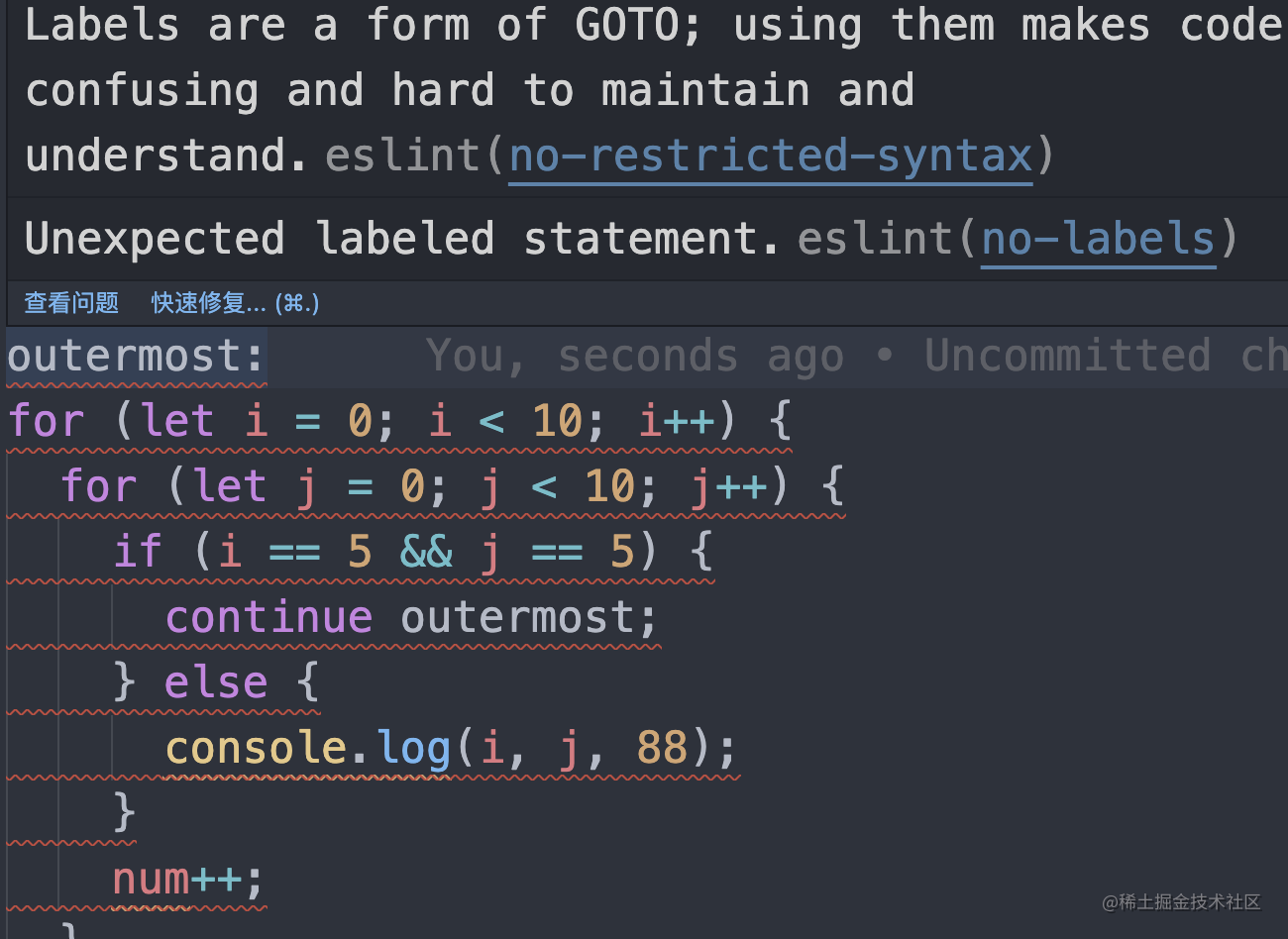Vue3又出新语法 到底何时才能折腾完？__前端__Vue.js__JavaScript

# 前言

``````<script setup>
import { ref, reactive } from 'vue'

const user = reactive({
name: '令狐冲',
age: 22,
gender: '男'
})
</script>
``````

``````<script setup>
import { ref } from 'vue'

}
</script>
``````

``````<script setup>
import { ref } from 'vue'

}
</script>
``````

`reactive`的弊端是不能解构，解构就会失去响应性：

``````<script setup>
import { reactive } from 'vue'

const user = reactive({
name: '令狐冲',
age: 22,
gender: '男'
})

// 这种写法通常达不到预期的效果
let { age } = user
age = 18
</script>
``````

``````<script setup>
import { reactive, toRefs } from 'vue'

const user = reactive({
name: '令狐冲',
age: 22,
gender: '男'
})

let { age } = toRefs(user)
age.value = 18
</script>
``````

# 第一波语法糖

``````<script>
export let title;

// 这将在“title”的prop属性更改时更新“document.title”
\$: document.title = title;

\$: {
console.log(`multiple statements can be combined`);
console.log(`the current title is \${title}`);
}
</script>
``````

``````let num = 0

outermost:
for (let i = 0; i < 10; i++) {
for (let j = 0; j < 10; j++) {
if (i == 5 && j == 5) {
continue outermost
} else {
console.log(i, j, 88)
}
num++
}
}

console.log(num) //95
````````````<script setup>

}
</script>
``````

# 第二波语法糖

``````<script setup>

}
</script>
``````

``````import { ref } from 'vue'

``````

``````<!-- 以前 -->
<script setup>
import { ref, computed } from 'vue'

const num = ref(1)
const num_10 = computed(() => num.value * 10)
</script>

<!-- 现在 -->
<script setup>
let num = \$ref(1)
const num_10 = \$computed(() => num * 10)
</script>
``````

`\$fromRefs`又是个啥呢？这玩意在之前没有啊！只听说过`toRefs`

``````<script setup>
import { fromRefs } from 'vue' // 这个API并不存在
import { toRefs } from 'vue' // 这个API倒是有 也就是只有 to 没有 from
</script>
``````

``````import { reactive } from 'vue'

const state = reactive({
x: 0,
y: 0
})

export default = (x = 0, y = 0) => {
state.x = x
state.y = y

}
``````

``````<script setup>
import { useXxx } form '../useXxx.js'

const { x, y } = useXxx(100, 200)

console.log(x.value, y.value)
</script>
``````

``````<script setup>
import { useXxx } form '../useXxx.js'

const { x, y } = \$fromRefs(useXxx(100, 200))

console.log(x, y)
</script>
``````

``````<script setup>

</script>
``````

## 改进版

``````<script setup>
import { ref } from 'vue'

</script>
``````

``````<script setup>
import { ref } from 'vue'

</script>
``````

``````<script setup>
import { ref } from 'vue'

</script>
``````

``````<script setup>
import { ref } from 'vue'

</script>
``````

# 第三波语法糖

• `ref`
• `computed`
• `shallowRef`
• `customRef`
• `toRef`

``````<script setup>
</script>
``````

``````<!-- 以前 -->
<script setup>
const props = defineProps({
type: Boolean,
default: true
}
}))

</script>

<!-- 现在 -->
<script setup>

</script>
``````